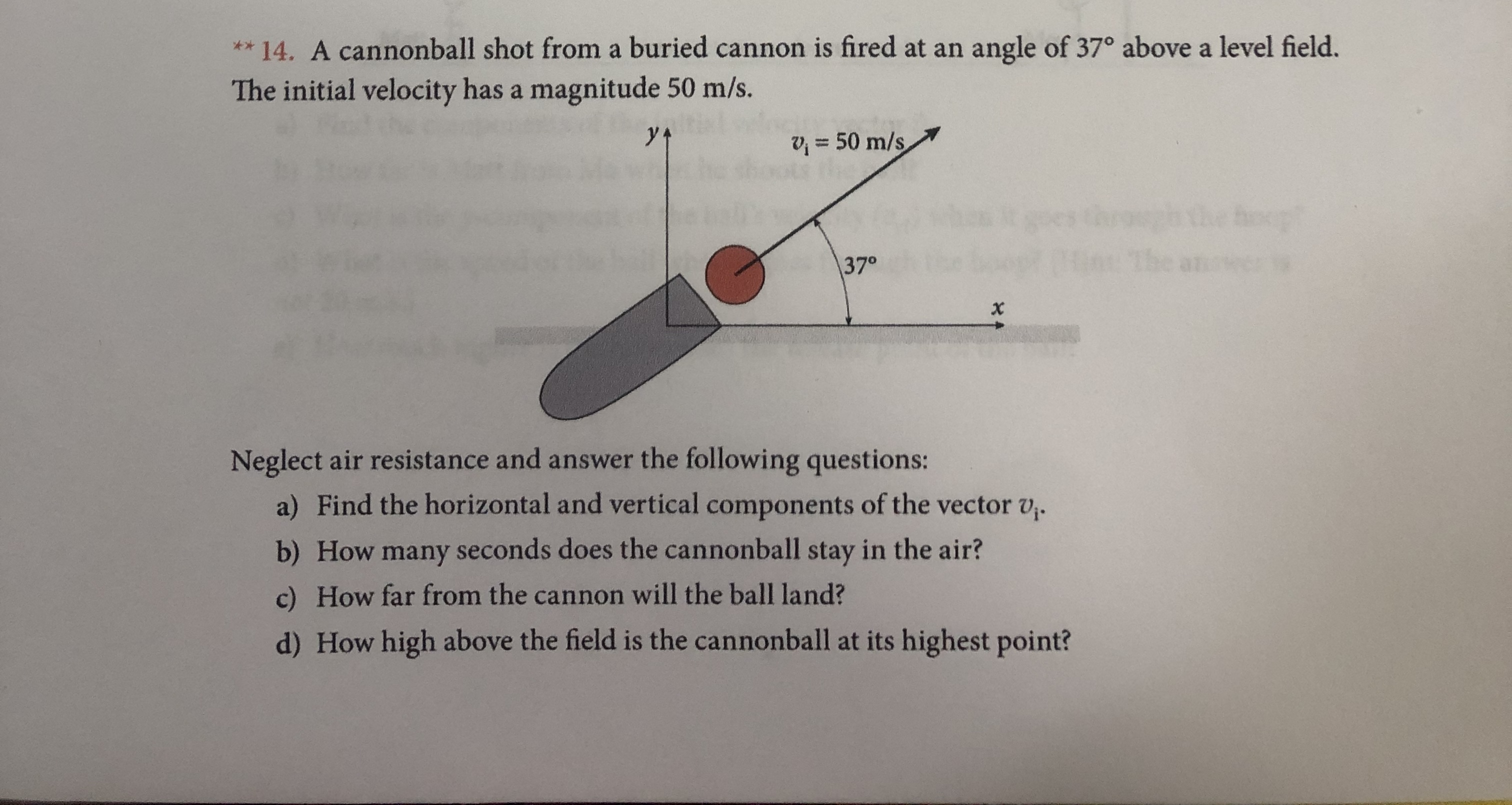# ** 14. A cannonball shot from a buried cannon is fired at anangle of 37° above a level field.The initial velocity has a magnitude 50 m/s.50 m/sthe oop5an:370xNeglect air resistance and answer the following questions:a) Find the horizontal and vertical components of the vector v.b) How many seconds does the cannonball stay in the air?c) How far from the cannon will the ball land?d) How high above the field is the cannonball at its highest point?

Question
114 viewshelp_outlineImage Transcriptionclose** 14. A cannonball shot from a buried cannon is fired at an angle of 37° above a level field. The initial velocity has a magnitude 50 m/s. 50 m/s the oop 5an: 370 x Neglect air resistance and answer the following questions: a) Find the horizontal and vertical components of the vector v. b) How many seconds does the cannonball stay in the air? c) How far from the cannon will the ball land? d) How high above the field is the cannonball at its highest point? fullscreen
check_circle

Step 1

The above question is an example of projectile motion.

Step 2

(a)

Let the horizontal component of the initial velocity u be denoted as ux.

Let the vertical component of the initial velocity u be denoted as uy.

From the figure the horizontal component of the velocity ux and the vertical component of the velocity uy are calculated as,

Step 3

Substitute 50 m/s for u in the above two equations and solve for...

### Want to see the full answer?

See Solution

#### Want to see this answer and more?

Solutions are written by subject experts who are available 24/7. Questions are typically answered within 1 hour.*

See Solution
*Response times may vary by subject and question.
Tagged in

### Kinematics